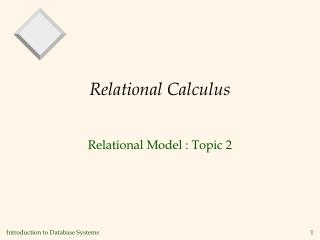DownloadDownload PresentationRelational Calculus

# Relational Calculus

Télécharger la présentation## Relational Calculus

- - - - - - - - - - - - - - - - - - - - - - - - - - - E N D - - - - - - - - - - - - - - - - - - - - - - - - - - -
##### Presentation Transcript

1. Relational Calculus Relational Model : Topic 2

2. Relational Calculus • Comes in two flavors: Tuple relational calculus (TRC) and Domain relational calculus(DRC). • Calculus has variables, constants, comparison ops, logical connectives and quantifiers. • TRC: Variables range over (i.e., get bound to) tuples. • DRC: Variables range over domain elements (= field values). • Both TRC and DRC are simple subsets of first-order logic. • Calculus expressions called formulas. • Answer tuple: assignment of constants to variables that makes formula evaluate to true.

3. Domain Relational Calculus • Query has the form: • Answer includes all tuples that • make the formula be true. • Formula is recursively defined, starting with • simple atomic formulas and building bigger • and better formulas using logical connectives.

4. DRC Formulas • Atomic formula: • , or X op Y, or X op constant • op is one of • Formula: • an atomic formula, or • , where p and q are formulas, or • , where variable X is free in p(X), or • , where variable X is free in p(X)

5. Example Instances • “Sailors”, “Reserves”, and “Boats” relations • Sailors(Id, Name, TechRating, Age) • Boats(Id, Name, Color) • Reserves(SailorId, BoatId, Date)

6. Find all sailors with a rating above 7 • The condition ensures that the domain variables I, N, T and A get bound to fields of the same Sailors tuple. • The term to the left of `|’ says that every tuple that satisfies T>7 is in the answer. • Modify this: • find all sailors who are older than 18 or have a rating under 9, and are called ‘Aaron’.

7. Find sailors rated > 7 who’ve reserved boat #103 • We have used as a shorthand for

8. Find sailors rated > 7 who’ve reserved a red boat

9. Find sailors who’ve reserved all boats • Find all sailors I such that for each 3-tuple either it is not a tuple in Boats or there is a tuple in Reserves showing that sailor I has reserved it.

10. ..... Find sailors who’ve reserved all boats (again!) • Simpler notation, same query. (Much clearer!) • To find sailors who’ve reserved all red boats:

11. Unsafe Queries • Unsafe query: Legal calculus, but an infinite number of answers! • e.g.,